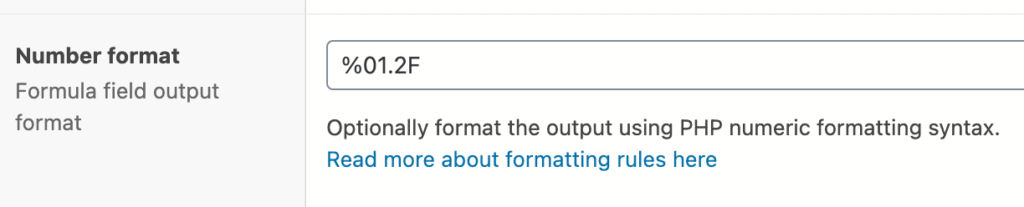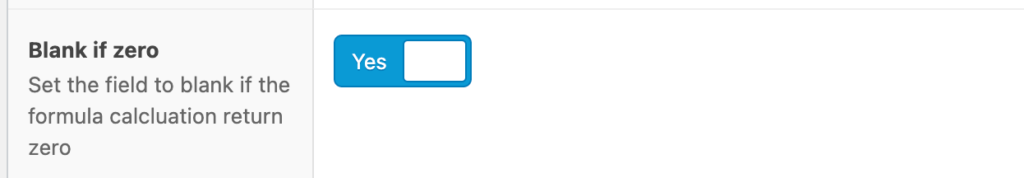### Categories

Calculated Fields for ACF supports formatting the formula output using a format string. The format string is passed to the PHP sprintf() function. So any format string that works with that function also works with this plugin. The format string is entered into the "Number format" in the field definition:

## Formatting rulesThe PHP formatting string consists of five different segments (well, actually six but in the case of this plugin, only five of them matters), percent sign, flags, width, precision, and type specifier put together like this:

%[flags][width][.precision]specifier

Percent sign %

The percent sign marks the beginning of a number format string. It always needs to be there to mark the beginning of a rule to format a number.

Flags (optional)

The flags are used to pad the resulting output if needed. It can tell you how to pad (left or right justify) and what character to use, usually zeros or spaces. The flags often only make sense if you also use the width described below.

Width

The minimum width of the resulting outputted string. You can use this to force the output to always have the same string length.

Precision

The number of decimals to use when the type is a floating-point number. You can use this to force a fixed number of decimals in the output.

Specifier

The specifier defies how to treat the number. Typically the most used values would be "d" for a normal integer and "F" for a floating-point number.

Read the full details about the different parts and how to combine them in the PHP documentation.

### Examples

Sometimes the easiest way to explain something is to use examples. Here are a few common format strings that you may find useful:

#### Integer examples

Format string Input Output Explanation
%d 12 12 Convert the input to an integer.
%d 12.33 12 Like above.
%5d 12 12 Like above. Pad the result with spaces so that the total output is 5 characters wide.
%05d 12 00012 Like above, but pad using zeros.

#### Floating-point examples

Format string Input Output Explanation
%F 12.123 12.123000 Convert the input to a floating-point number with 6 decimal places.
%F 12.123456789 12.123457 Like above.
%0.2F 12.12345 12.12 Like above, but with two decimal places.
%0.2F 12 12.00 Like above, not that there are always two decimal places.

#### Currency examples

Format string Input Output Explanation
$%0.2F 12$12.00 Note that anything before the percent sign will be outputted "as is". This can be used to add a currency symbol.
%0.2F SEK 12 12.00 SEK Like above, but with additional characters after the type specification. These are also outputted as is.

## Blank if zero

It’s also possible to omit output if the formula returns zero (0). To do this, toggle the "Blank if zero" field to Yes.